# Programming Language Semantics Axiomatic Semantics Chapter 6 Motivation

• Slides: 30Programming Language Semantics Axiomatic Semantics Chapter 6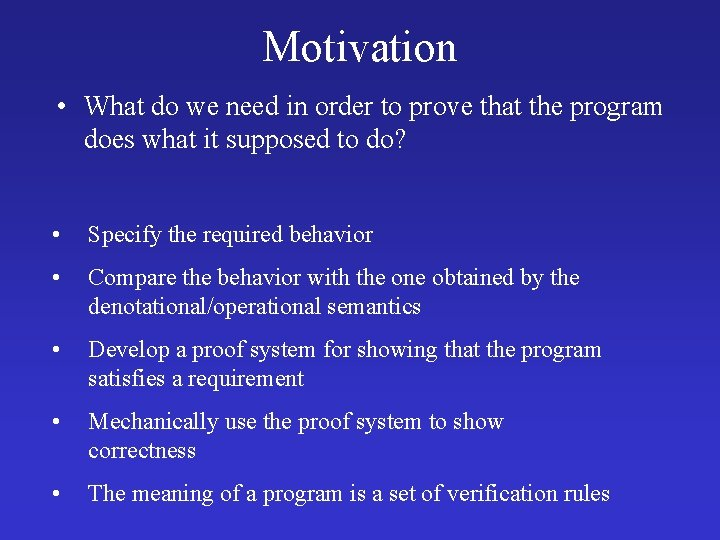Motivation • What do we need in order to prove that the program does what it supposed to do? • Specify the required behavior • Compare the behavior with the one obtained by the denotational/operational semantics • Develop a proof system for showing that the program satisfies a requirement • Mechanically use the proof system to show correctness • The meaning of a program is a set of verification rules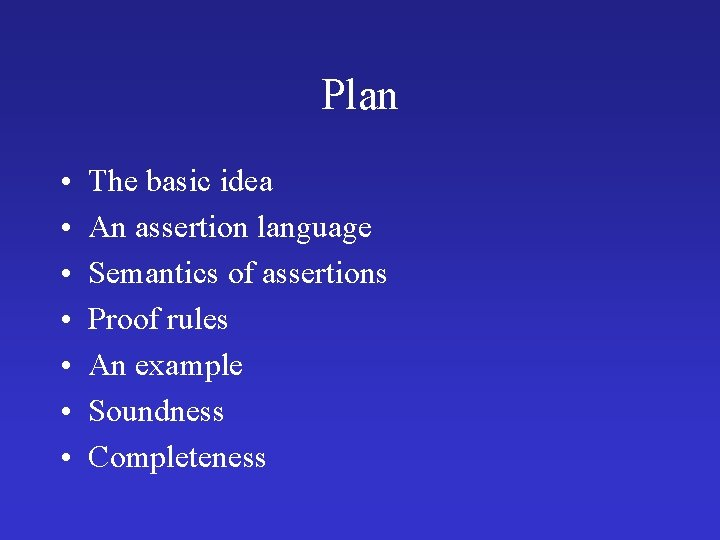Plan • • The basic idea An assertion language Semantics of assertions Proof rules An example Soundness Completeness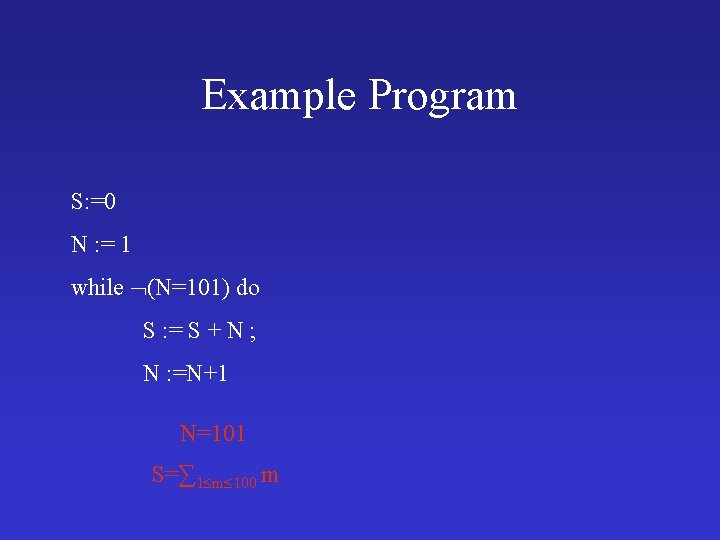Example Program S: =0 N : = 1 while (N=101) do S : = S + N ; N : =N+1 N=101 S=∑ 1 m 100 m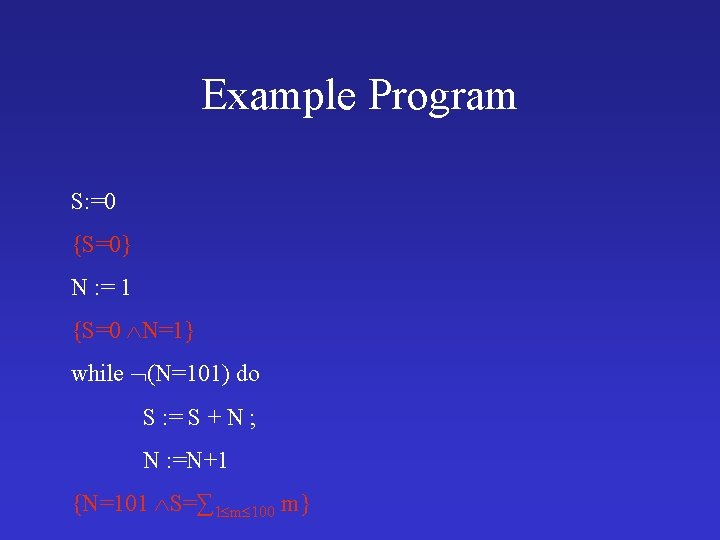Example Program S: =0 {S=0} N : = 1 {S=0 N=1} while (N=101) do S : = S + N ; N : =N+1 {N=101 S=∑ 1 m 100 m}Example Program S: =0 {S=0} N : = 1 {S=0 N=1} while (N=101) do S : = S + N ; N : =N+1 {N=101 S=∑ 1 m 100 m}Example Program S: =0 {S=0} N : = 1 {S=0 N=1} while {1 N 101 S=∑ 1 m N-1 m} (N=101) do S : = S + N ; {1 N < 101 S=∑ 1 m N m} N : =N+1 {N=101 S=∑ 1 m 100 m}Partial Correctness • {P}c{Q} – P and Q are assertions (extensions of Boolean expressions) – c is a command – For all states which satisfies P, if the execution of c from state terminates in state ’, then ’ satisfies Q • {true}while true do skip{false}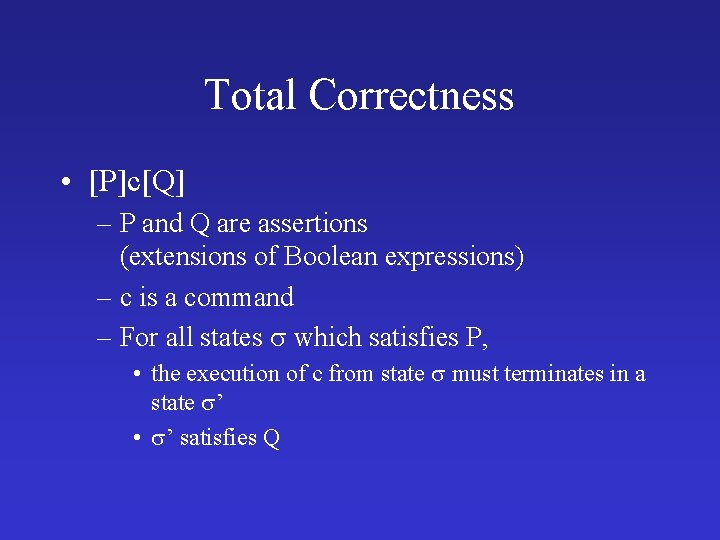Total Correctness • [P]c[Q] – P and Q are assertions (extensions of Boolean expressions) – c is a command – For all states which satisfies P, • the execution of c from state must terminates in a state ’ • ’ satisfies QFormalizing Partial Correctness • A – A is true in • {P} c {Q} – , ’ ∑. ( P & <c, > ’ ) ’ Q – ∑. ( P & C c ) C c Q • Convention for all A A • , ’ ∑. P C c Q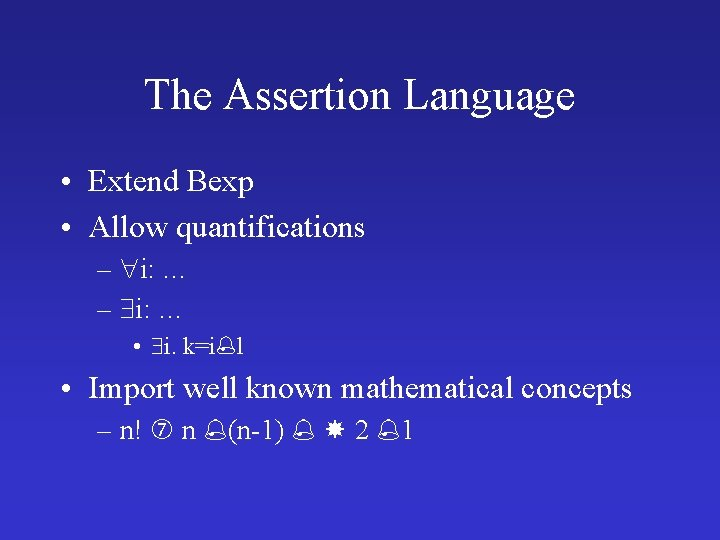The Assertion Language • Extend Bexp • Allow quantifications – i: … • i. k=i l • Import well known mathematical concepts – n! n (n-1) 2 1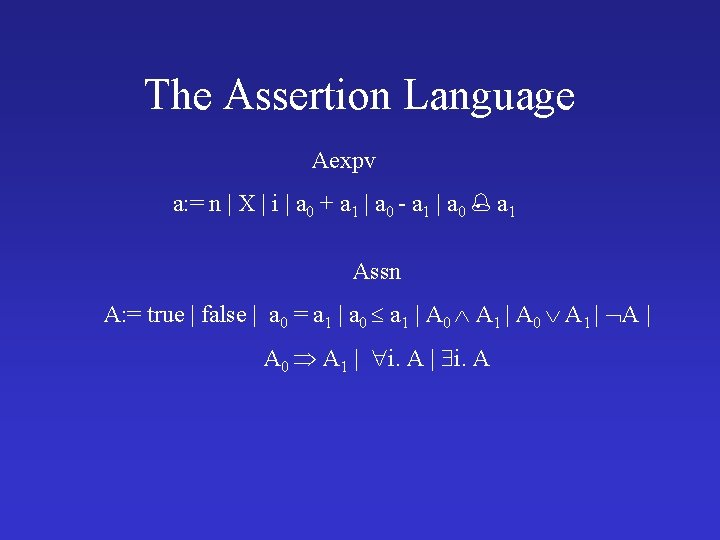The Assertion Language Aexpv a: = n | X | i | a 0 + a 1 | a 0 - a 1 | a 0 a 1 Assn A: = true | false | a 0 = a 1 | a 0 a 1 | A 0 A 1 | i. AExample while (M=N) do if M N then N : = N – M else M : = M - NFree and Bound Variables • An integer variable is bound when it occurs in the scope of a quantifier • Otherwise it is free • Examples i. k=i L (i+100 77) i. j+1=i+3) FV(n) = FV(X) = FV(i) = {i} FV(a 0 + a 1)=FV(a 0 -a 1)=FV(a 0 a 1 ) = FV(a 0) FV(a 1) FV(true)=FV(false)= FV(a 0 = a 1)=FV(a 0 a 1)= FV(a 0) FV(a 1) FV(A 0 A 1)=FV(A 0 A 1)= FV(A 0) FV(A 1) FV( A)=FV(A) FV( i. A)= FV(A) {i}Substitution • Visualization of an assertion A ---i--- • Consider a “pure” arithmetic expression A[a/i] ---a--n[a/i] = n X[a/i]=X i[a/i] = a j[a/i] = j (a 0 + a 1)[a/i] = a 0[a/i] + a 1/[a/i] (a 0 - a 1)[a/i] = a 0[a/i] – a 1[a/i] (a 0 a 1 )[a/i]= a 0[a/i] a 1[a/i]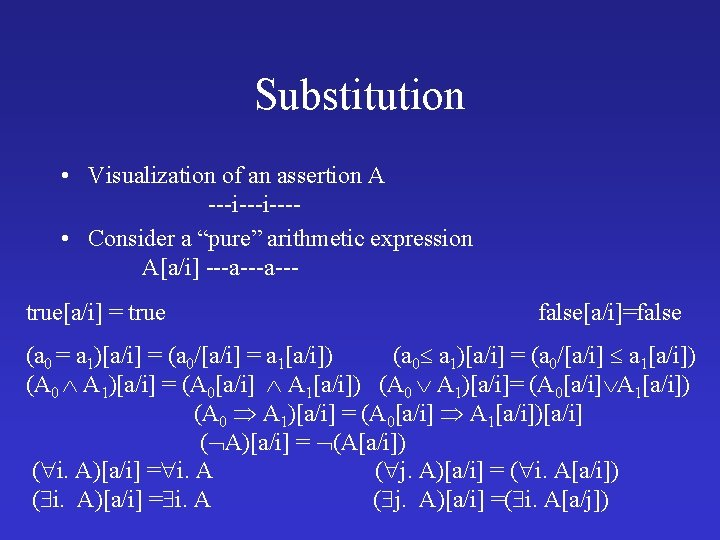Substitution • Visualization of an assertion A ---i--- • Consider a “pure” arithmetic expression A[a/i] ---a--true[a/i] = true false[a/i]=false (a 0 = a 1)[a/i] = (a 0/[a/i] = a 1[a/i]) (a 0 a 1)[a/i] = (a 0/[a/i] a 1[a/i]) (A 0 A 1)[a/i] = (A 0[a/i] A 1[a/i]) (A 0 A 1)[a/i]= (A 0[a/i] A 1[a/i]) (A 0 A 1)[a/i] = (A 0[a/i] A 1[a/i])[a/i] ( A)[a/i] = (A[a/i]) ( i. A)[a/i] = i. A ( j. A)[a/i] = ( i. A[a/i]) ( i. A)[a/i] = i. A ( j. A)[a/i] =( i. A[a/j])Location Substitution • Visualization of an assertion A ---X--- • Consider a “pure” arithmetic expression A[a/X] ---a---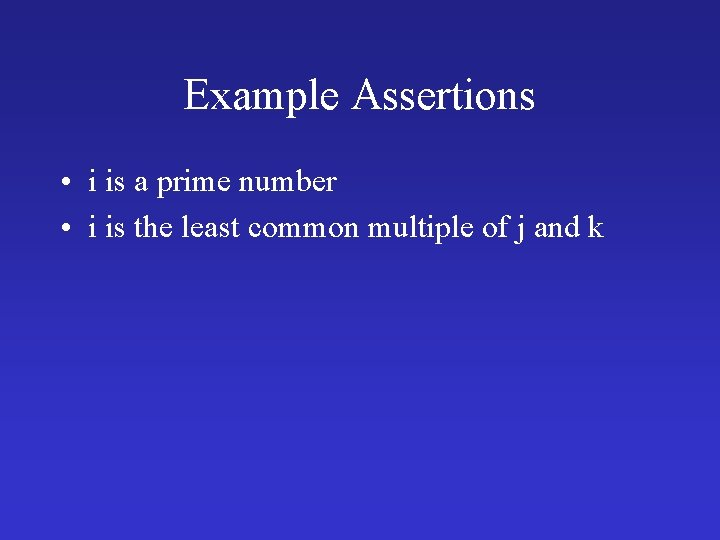Example Assertions • i is a prime number • i is the least common multiple of j and kSemantics of Assertions • An interpretation I: intvar N • The meaning of Aexpv – – – Av n I =n Av X I = (X) Av i I = I(i) Av a 0+a 1 I = Av a 0 I +Av a 1 I … • For all a Aexp states and Interpretations I – A a =Av a I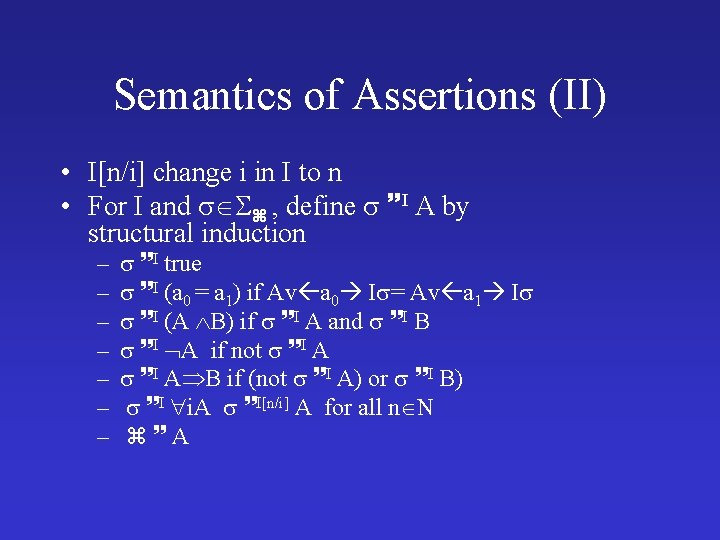Semantics of Assertions (II) • I[n/i] change i in I to n • For I and , define I A by structural induction – – – – I true I (a 0 = a 1) if Av a 0 I = Av a 1 I I (A B) if I A and I B I A if not I A B if (not I A) or I B) I i. A I[n/i] A for all n N AProposition 6. 4 For all b Bexp states and Interpretations I B b = true iff I b B b = false iff not I b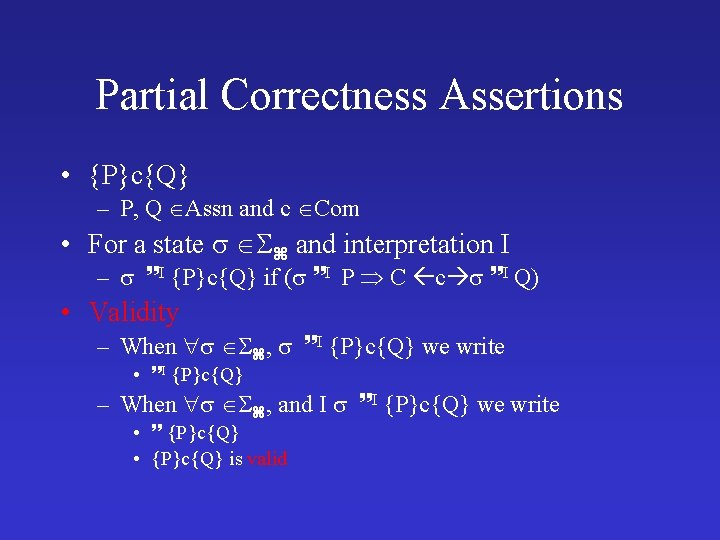Partial Correctness Assertions • {P}c{Q} – P, Q Assn and c Com • For a state and interpretation I – I {P}c{Q} if ( I P C c I Q) • Validity – When , I {P}c{Q} we write • I {P}c{Q} – When , and I I {P}c{Q} we write • {P}c{Q} • {P}c{Q} is validThe extension of an assertion AI { | I A }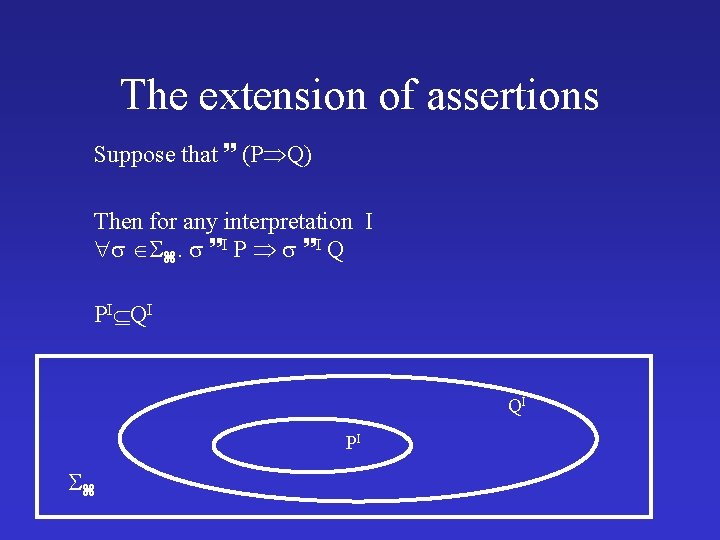The extension of assertions Suppose that (P Q) Then for any interpretation I . I P I Q PI QI QI PI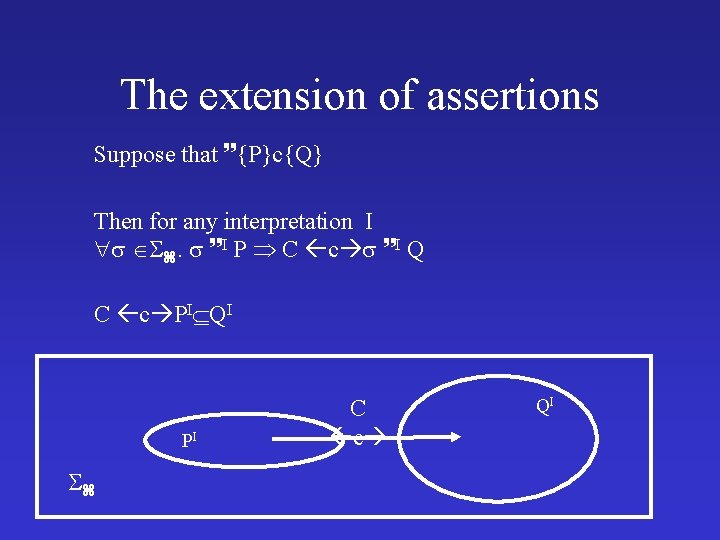The extension of assertions Suppose that {P}c{Q} Then for any interpretation I . I P C c I Q C c PI QI PI C c QI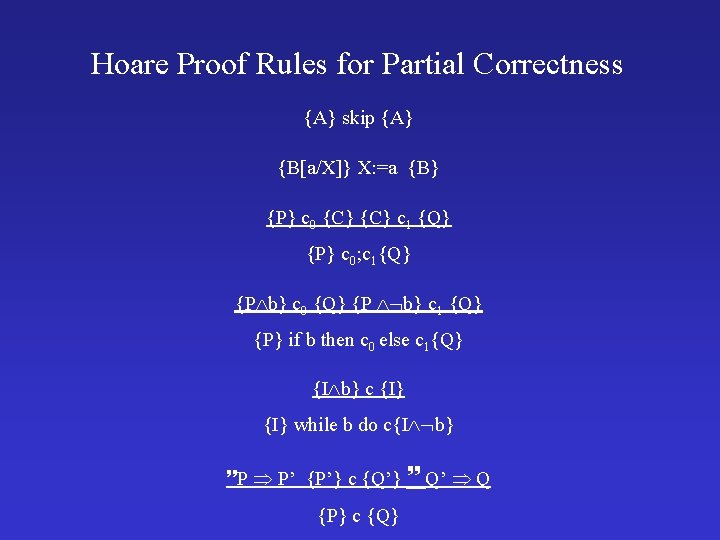Hoare Proof Rules for Partial Correctness {A} skip {A} {B[a/X]} X: =a {B} {P} c 0 {C} c 1 {Q} {P} c 0; c 1{Q} {P b} c 0 {Q} {P b} c 1 {Q} {P} if b then c 0 else c 1{Q} {I b} c {I} while b do c{I b} P P’ {P’} c {Q’} Q’ Q {P} c {Q}Example while X > 0 do Y : = X Y; X : = X – 1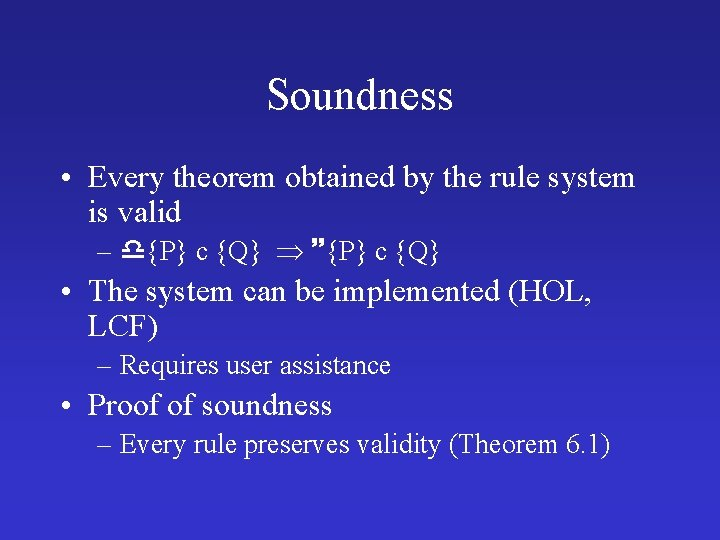Soundness • Every theorem obtained by the rule system is valid – {P} c {Q} • The system can be implemented (HOL, LCF) – Requires user assistance • Proof of soundness – Every rule preserves validity (Theorem 6. 1)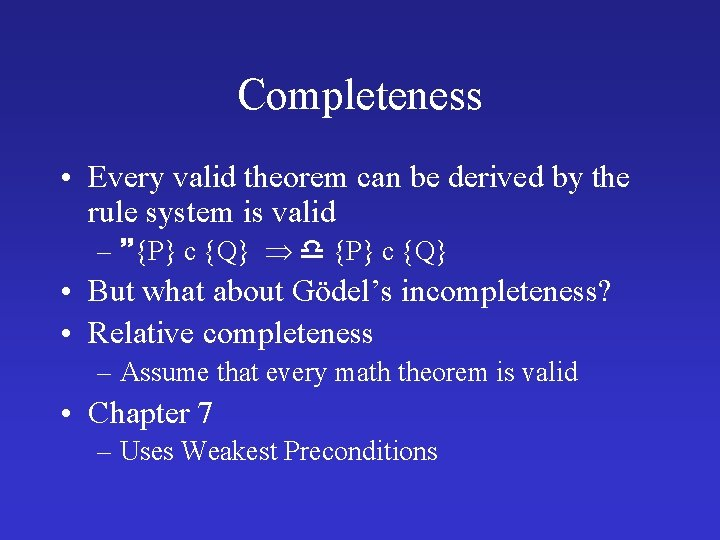Completeness • Every valid theorem can be derived by the rule system is valid – {P} c {Q} • But what about Gödel’s incompleteness? • Relative completeness – Assume that every math theorem is valid • Chapter 7 – Uses Weakest Preconditions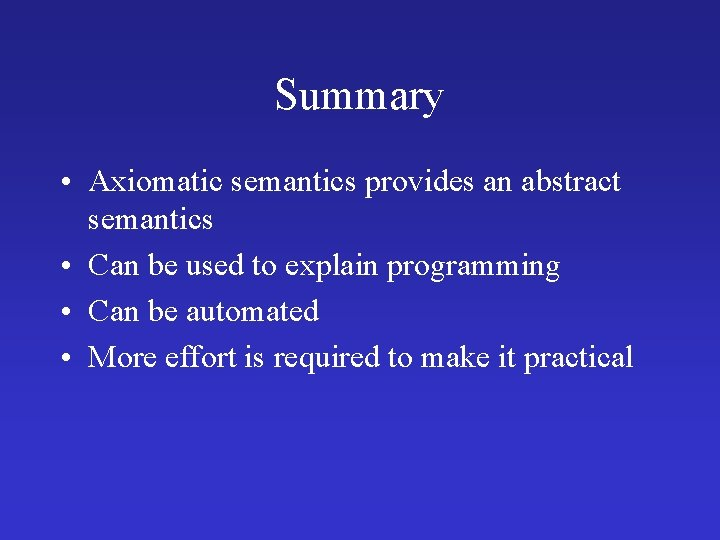Summary • Axiomatic semantics provides an abstract semantics • Can be used to explain programming • Can be automated • More effort is required to make it practical